Test: Electromagnetic Waves- 2

# Test: Electromagnetic Waves- 2 - Electrical Engineering (EE)

Test Description

## 20 Questions MCQ Test Topicwise Question Bank for Electrical Engineering - Test: Electromagnetic Waves- 2

Test: Electromagnetic Waves- 2 for Electrical Engineering (EE) 2023 is part of Topicwise Question Bank for Electrical Engineering preparation. The Test: Electromagnetic Waves- 2 questions and answers have been prepared according to the Electrical Engineering (EE) exam syllabus.The Test: Electromagnetic Waves- 2 MCQs are made for Electrical Engineering (EE) 2023 Exam. Find important definitions, questions, notes, meanings, examples, exercises, MCQs and online tests for Test: Electromagnetic Waves- 2 below.
Solutions of Test: Electromagnetic Waves- 2 questions in English are available as part of our Topicwise Question Bank for Electrical Engineering for Electrical Engineering (EE) & Test: Electromagnetic Waves- 2 solutions in Hindi for Topicwise Question Bank for Electrical Engineering course. Download more important topics, notes, lectures and mock test series for Electrical Engineering (EE) Exam by signing up for free. Attempt Test: Electromagnetic Waves- 2 | 20 questions in 60 minutes | Mock test for Electrical Engineering (EE) preparation | Free important questions MCQ to study Topicwise Question Bank for Electrical Engineering for Electrical Engineering (EE) Exam | Download free PDF with solutions
 1 Crore+ students have signed up on EduRev. Have you?
Test: Electromagnetic Waves- 2 - Question 1

### The propagation constant γ for a material with μr = 1, εr = 8, σ = 0.25 pS/m and having the wave frequency of 1.6 MHz would be approximately equal to

Detailed Solution for Test: Electromagnetic Waves- 2 - Question 1

Given,
σ = 0.25 pS/m; μr = 1; εr = 8;
f = 1.6 x 106 Hz
Now,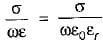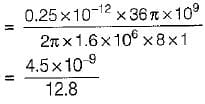or,   σ/ωε = 0.3515 x 10-9 ≈ 0
or, σ  ≈ 0
Since σ = 0, therefore the material will behave as a perfect dielectric (α = 0).
∴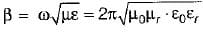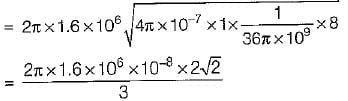∴ Propagation constant,
γ = α + jβ = 0 + j 9.471 x 10-2
≈ j.095 m-1

Test: Electromagnetic Waves- 2 - Question 2

### What is the skin depth in copper at 10 GHz? Assuming conductivity of copper σ = 5.8 x 107 mhos/m and permeability equal to that of free space.

Detailed Solution for Test: Electromagnetic Waves- 2 - Question 2

Skin depth,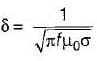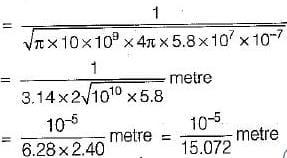= 0.66 x 10-6 m = 0.66μm

Test: Electromagnetic Waves- 2 - Question 3

### The velocity of a plane wave in a lossless medium having a relative permittivity of 4 and relative permeability of unity would be

Detailed Solution for Test: Electromagnetic Waves- 2 - Question 3

For a lossless or perfect medium, velocity of plane wave is given by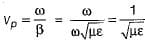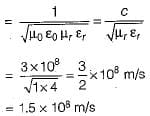Test: Electromagnetic Waves- 2 - Question 4

An elliptically polarized wave travelling in the +z direction in air has x and y components
Ex = 2 sin(ωt - βz) V/m;
Ey = 4 sin(ωt - βz + 75°) v/m
The average power per unit area conveyed by the wave is

Detailed Solution for Test: Electromagnetic Waves- 2 - Question 4

The average power per unit area is equal to the Poynting vector, the magnitude of which is given by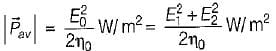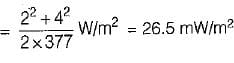Test: Electromagnetic Waves- 2 - Question 5

Consider the following statements:
1. A wave whose phase is constant over a set of planes is called plane wave.
2. A wave whose phase is constant while magnitude is variable is called uniform plane wave.
3. The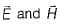fields are non-uniform over plane surfaces.
4. Earth is considered as good conductor in one part of the radio frequency range and as dielectric in other frequency range.
Which of the above statements is/are not correct?

Detailed Solution for Test: Electromagnetic Waves- 2 - Question 5
• A wave whose phase and magnitude both are constant is called uniform plane wave. Hence, statement-2 is not correct.
•  A uniform plane is one in whichlie in a plane and have the same value everywhere in that plane at any fixed instant.
• Thefields are uniform over plane surfaces. Hence, statement-3 is also not correct.
Test: Electromagnetic Waves- 2 - Question 6

Assertion (A): Displacement current directly passes through the capacitor.
Reason (R): The current through a capacitive element is called displacement current.

Detailed Solution for Test: Electromagnetic Waves- 2 - Question 6

Assertion is not correct because it is not the displacement current which directly passes through a capacitor but it is only apparant current representing the rate at which the flow of charge takes place from one plate of the capacitor to the other plate.

Test: Electromagnetic Waves- 2 - Question 7

In a homogeneous, isotropic, linear and stationary media, a plane electromagnetic wave has the following characteristics.

Detailed Solution for Test: Electromagnetic Waves- 2 - Question 7

In a homogeneous, isotropic, linear and stationary media, ∇·E = ρ = 0 and both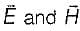are transverse.

Test: Electromagnetic Waves- 2 - Question 8

Assertion (A): The distance to which a wave penetrates a conductor is known as depth of penetration.
Reason (R): The depth of penetration (δ) is defined as that depth in which the wave has been attenuated to ‘e’ percent of its original value.

Detailed Solution for Test: Electromagnetic Waves- 2 - Question 8

The depth of penetration (δ) is defined as that depth in which the wave has been attenuated to ‘1/e’ percent (37%) of its original value. Hence, reason is not a correct statement.

Test: Electromagnetic Waves- 2 - Question 9

The polarization of a dielectric is given by

Test: Electromagnetic Waves- 2 - Question 10

In a travelling electrom agnetic wave, E and H vector fields are

Test: Electromagnetic Waves- 2 - Question 11

A wave is incident normally on a good conductor. If the frequency of a plane electromagnetic wave increases four times, the skin depth, will

Detailed Solution for Test: Electromagnetic Waves- 2 - Question 11

Skin depth (δ)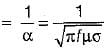∴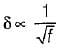When the frequency is increased 4-times, then skin depth will become half.

Test: Electromagnetic Waves- 2 - Question 12

The electric field of a travelling wave is given by 100 cos(109t - 4x). The velocity and the wavelength are respectively.

Detailed Solution for Test: Electromagnetic Waves- 2 - Question 12

Given,
E = 100 cos (109t - 4x)
or, E = E0 cos (ωt - βx)
and β = 4
Thus, ve lo city of propag ation is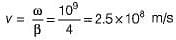and wavelength,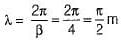Test: Electromagnetic Waves- 2 - Question 13

In a dielectric-conductor boundary (interface), the tangential component of electric field is

Test: Electromagnetic Waves- 2 - Question 14

Which of the following statements is not true of waves in general?

Detailed Solution for Test: Electromagnetic Waves- 2 - Question 14

A wave can’t be a function of time only. It is function of both space and time.

Test: Electromagnetic Waves- 2 - Question 15

When an EM wave is incident on a dielectric, it is

Test: Electromagnetic Waves- 2 - Question 16

Poynting vector gives

Detailed Solution for Test: Electromagnetic Waves- 2 - Question 16

The quantity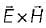is known as Poynting vector, measured in watt/m2.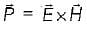Poynting vector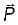represents the instantaneous power density vector associated with the EM field at a given point, in other words, it gives rate of energy flow.

Test: Electromagnetic Waves- 2 - Question 17

Match List-I with List-II and select the correct answer using the codes given below the lists:
List-I
A. Free space
B. Lossless dielectrics
C. Lossy dielectrics
D. Good conductors
List-lI
1. σ ≠ 0, ε = ε0εr, μ = μ0μr
2. σ = 0, ε = ε0, μ = μ0
3. σ ≈ ∞, ε = ε0, μ = μ0μr
4. σ = 0, ε = ε0εr, μ = μ0μr

Codes:
A B C D
(a) 4 2 3 1
(b) 2 4 1 3
(c) 4 2 1 3
(d) 2 4 3 1

Test: Electromagnetic Waves- 2 - Question 18

Match List-I (EM phenomena) with List-ll (Uses) and select the correct answer using the codes given below the lists:
List - I
A. Cosmic rays
D. Gamma rays
List - II
1. Sterilization
2. Cancer therapy
3. Astronomy
4. Photography
Codes:
A B C D
(a) 4 3  2 1
(b) 3 4  1 2
(c) 1 2  3 4
(d) 3 1 4  2

Test: Electromagnetic Waves- 2 - Question 19

A lossy dielectric has an intrinsic impedance of 200∠30° Ω at a particular frequency. If, at that frequency, the plane wave propagating through the dielectric has the magnetic field component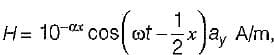the skin depth will be

Detailed Solution for Test: Electromagnetic Waves- 2 - Question 19

Given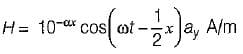Here,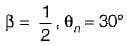Now,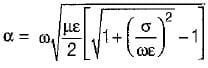and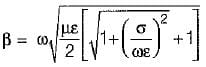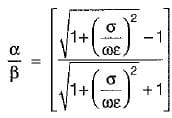But,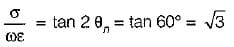Hence,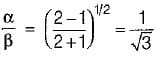or,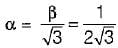So, skin depth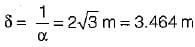Test: Electromagnetic Waves- 2 - Question 20

What is the major factor for determining whether a mediam is free space, lossless dielectric, or good conductor?

## Topicwise Question Bank for Electrical Engineering

211 tests
Information about Test: Electromagnetic Waves- 2 Page
In this test you can find the Exam questions for Test: Electromagnetic Waves- 2 solved & explained in the simplest way possible. Besides giving Questions and answers for Test: Electromagnetic Waves- 2, EduRev gives you an ample number of Online tests for practice

211 tests